Home | Study Links | Rhymes |

## Primes and Patterns

Primes are the atoms and basic building blocks of mathematics. The challenge for hundreds of years
has been to decide whether a given number is a prime or not, and to find a pattern in the distribution
of the primes. I shared my ideas and findings February 2014 at Yukon College (YRC-dept).Instead of looking for a pattern in the prime numbers, I began looking for it in the non-prime numbers.When lining up odd and even numbers in this manner, I observed that
the #3 frequency contained a repeating pattern at regular intervals of 6.
Non-prime numbers are blue and green, and prime numbers are orange.The spacings between the twin prime sets such as 11/13 and 17/19, consist of multiples of 3.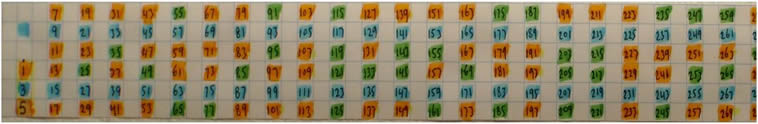When lining up the sections horizontally, a zigzag pattern forming a frequency wave and consisting
entirely of non-prime numbers became apparent, with numbers bouncing from 3 to 9 to 15- etc. at
regular intervals of 6. The appearance of this strip pattern brought to mind objects such as, a ribbon,
tube, high-speed camera, movie filmstrip, and a railroad track. (vertical spacing's for easier viewing)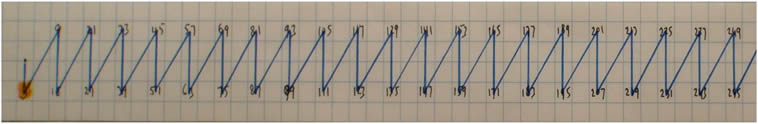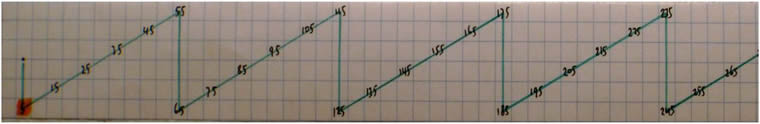The finding of #3-frequency, was quickly followed by the #5-frequency pattern.Chart B shows twin primes, and the lines separating them suggests measured spectral rays
and time lines in the electromagnetic spectrum, which range from short gamma to long frequency
radio waves. The spacing’s between them are multiples of 3 and can be measured accurately.

From here I continued with 20 or so additional sequential frequency waves.
As the prime number values increase, new frequency waves are produced!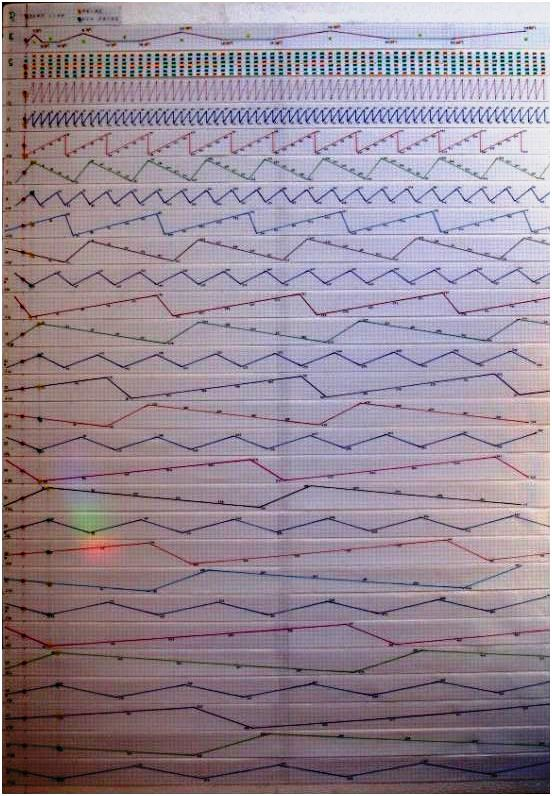Non-prime numbers consist of electromagnetic spectrum frequency waves, like finely
woven threads of fabric, and prime numbers represent the spaces in that net or grid.
Interestingly, while we in the west generally look for patterns in the stars,
Australian aboriginals look for patterns in the spaces between the stars.

Prime numbers are like musical notes and non-prime numbers describe their vibrational wave
patterns in an exact and predictable manner. To know the note, you must first hear the sound!The #1 frequency wave includes all of the numbers on the strip, both prime and non-prime
numbers. All other frequency waves consist of non-prime numbers only, except for the very
first number beginning each prime frequency wave! Frequencies 3-5-7, the first few primes,
cover most of the non-prime numbers on the entire strip. (All grid lines represent even numbers.)

The #1 ultra high frequency wave contains, or consists of, the entire EMF spectrum.

The numbers belonging to the #3-frequency as mentioned earlier, occur at intervals of 6.
The #5-frequency has intervals of 10, number 7 has 14, number 9 has 18, and so on.
It increases by 4 each time. For example 3-9-15…, 5-15-25…, 7-21-35…, 9-27-45… etc.
(when the vertical spacing's for easier viewing are eliminated, the increases are 2)As the frequency numbers increase in size, meaning the very first number beginning each frequency
wave after the zero point as represented by the red pins, their wave segments stretch accordingly.
Moreover, the frequencies and their segments or wave sections, match each other in specific ways.
For example, five #7-frequency segments = seven #5-frequency wave segments. Five #11-frequency
segments = eleven #5-frequency wave segments. Seven #11-frequency segments = eleven
#7-frequency wave segments. Etc. The frequencies contain many interesting patterns.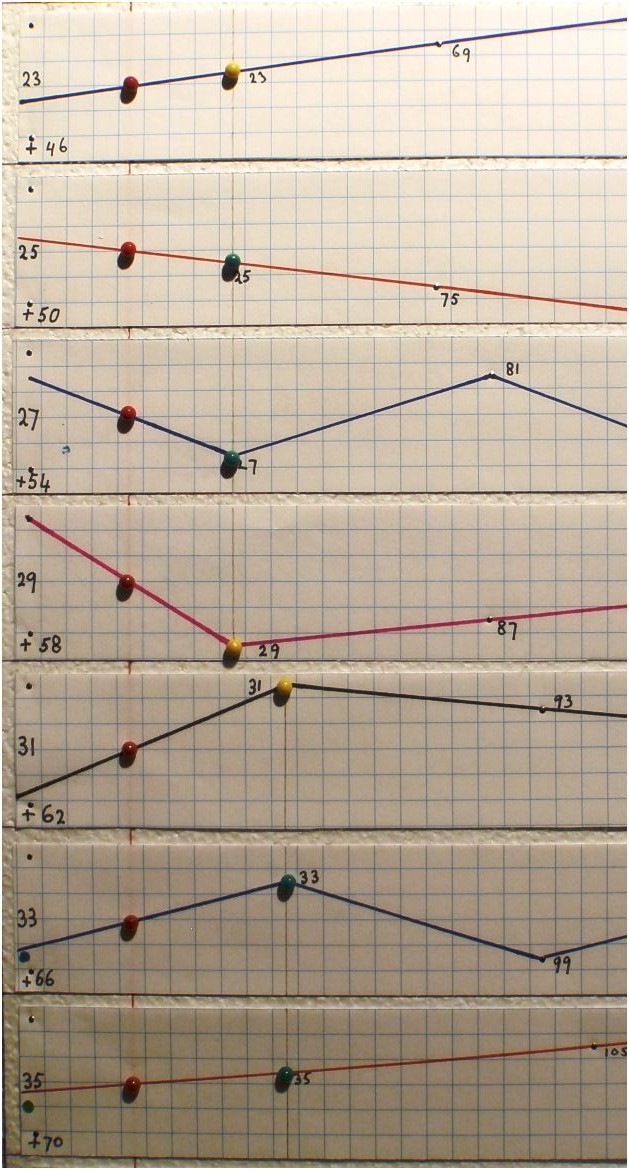By knowing the shape and arrangements of the frequency waves, one can predict prime numbers a
million miles down the strip. Nevertheless, how to know exactly at which points along the strip new
frequencies are required, is explained with this important discovery, I willl call it the "pyramid strip".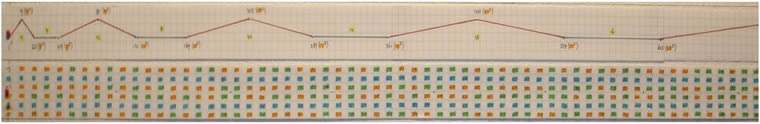The Pyramid strip overlays the 'C' or #1- frequency strip. It determines exactly where,
and how far along the strip, the various frequency insertion points are located,
depending on the need to know the size of the numbers.
The pyramids stretch with predictable increases, while their heights remain unchanged.Again, the #3-frequency covers all other related frequencies such as 9-15-21-27-etc, so that after
the first small pyramid 1-9-25 no other pyramid peaks are required, and only the base line numbers
are used from here on. The spacing ratio for the bottom line is 12-52-72-112-132-172-192-232- 252 etc.,
(4-2-4-2-4-2-etc). Alternatively as ratios 2-1-2-1-2-1 or 1-0-1-0-1-0 etc.

However, 252 and all numbers that are part of the prime 5 frequency sitting on these
ratio points could also be eliminated, but the consistent 4-2-4-2-4 pattern would be lost.
This idea continues with the next frequency number 7 and 492 for example.The pyramid's base line maintains the same consistent level, 3 squares up and 4 down.To demonstrate how perfect and orderly the prime numbers (colored orange), are encapsulated,
carried and fit untouched between all of the frequency waves, I made a #1-frequency strip board
with all the prime numbers marked on it in their proper place, and transparent strips for the first
several frequencies that will overlay it.Begin by placing the #3 frequency on the #1 board,
which fills in all of the non-prime numbers to 25 (52).The #5 frequency fills in the non-prime numbers to number 49 (72),
leaving only prime numbers marked as orange.Frequency #7 to 121 (112)Frequency #11 to 169 (132)Frequency #13 to 289 (172)

Place frequency #17 and continue like this.

It effectively separates and sifts out the prime numbers from the non-prime numbers.
Example: At number 25 (52) enter frequency #5, at number 49 (72) enter frequency #7, and so on.

Imagine a train moving along a train track and passing known and predetermined stops along
the way. At each stop a new frequency is engaged to cover all non-prime numbers on the strip
up to the next stop and ahead of the train. As the distances between stops increase continually
the train can speed up since there are fewer stops and frequencies required. Again, distance and
speed increases but the frequency numbers decrease. In other words, placing progressively longer
sections of track ahead of a faster and faster moving train.
Compare to the infinity π line and a thought-provoking postulation by David.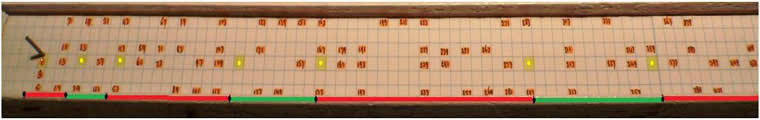The measurements for the stops along the primes-board increase at predictable intervals:
Red = 1”-3”-5”-7”-9”-etc, and green sections 1”-2”-3”-4”-5”-etc. (The strip has 4 squares per inch)

Imagine this primes-board represents a line of sight looking out into the universe from 0 (the peg),
where from that position it appears to be expanding same as the prime numbers, at an increasing
but predictable rate. Logically, an expanding universe already consists of pre-existing ever-growing
numbers, frequencies, patterns, and primes.What Riemann observed in his mathematics were the peaks and valleys of frequency waves with
a common line running through them, the zero line. (Image from the The story of Math DVD)The Frequency waves are separated for display purposes, and the zeros lie in a straight line.

Compare the vertical sections such as section 7 to 17 on the strip below, with the separated
frequency layouts on the board. Observe how the frequency waves are shaped, and how the 6
frequency line sections merge to points on the left side of the so-called zero line. Those points
west of the zero line show-increasing intervals of 3”. E.g., frequency lines 11 and 13 merge at 3”
west of the zero line and lines 23 and 25 merge 6” west of the zero line. Connecting those points
on the left side of the zero line will produce a line intersecting with the zero line at -6, and
tapering away from the zero line at 18.4°. The triangle consists of angles 90°, 71.6°, and 18.4°.
Again, to this point the vertical spacing's were added for easier viewing!The #1-Frequency correctly displayed without vertical spacing's. (triangle = 90°, 80.5°, 9.5°)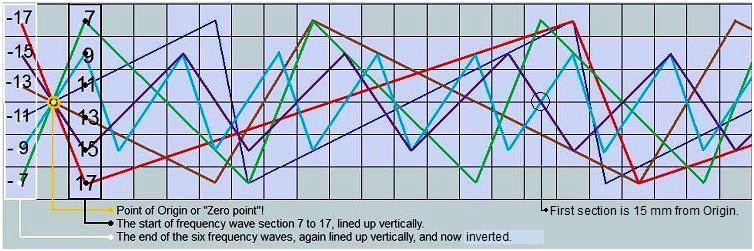From the strip board pin (zero point, "infinity"), and this example of six frequencies (section 7 to 17),
it shows that all of the frequencies emanate from a single source reversal point!
Therefore, section 7 to 17 as the start of those 6 frequencies, and section -17 to -7 mirroring those
frequency waves, occur simultaneously.

The simultaneous opposing positions between entangled quantum particles, i.e. particles of light.In flat 2-d form all frequencies emanate from a single source zero point. However, due to twisting
the flat 2-d into the 3-d form, it shows that in tubular 3-d form there appear to be two zero points.
However, the real point of origin is the center of the tube. The point of Origin or infinity point is
the source of all spiraling frequencies, like the center of a pie cut in 6 even slices!
It is the midpoint between positive and negative numbers, the midpoint of image reversal in a lens,
or the point between two realities.

The distance the 6 frequency waves (7 to 17) are from the Origin before they line up vertically
like they did at the start even though they are now inverted, is 382882.5 mm x 2= 765765 mm.
(Squares are 1 mm)

(The LCM is 7 x 9 x 11 x 13 x 15 x 17= 2297295 / 6= 382882.5)
1 section - 11 x 13= 143 mm-----------x 53550 = 765765 (143 mm from point of Origin)
9 sections - 9 x 15= 135 mm-- 15 mm x 51051 = 765765 (15 mm from point of Origin)
1 section  - 7 x 17= 119 mm------------x 64350 = 765765 (119 mm from point of Origin)Like increasing ripples in a circular pond for the subsequent wave sections such as 19-29, 31-41 etc.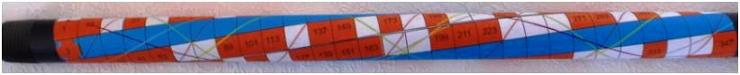Frequencies accurately shown as tubular, circular and spiralling, instead of flat and straight.
And as conjectured earlier, the #1 ultra high frequency wave as represented by the tube
contains the entire EMF spectrum.

In the Netherlands when someone dies, they have an interesting saying, "de pijp uit gaan".
Translated it says, "exiting the tube". Traveling from entry point to exit point and over again?
It seems so according to the mathematical model described here, because the perfect
arrangement of primes, non-primes and frequencies exist in this tubular model as shown.Frequency #3 appears to have stronger bonds than the other frequencies.A connection to this verse perhaps: "Or ever the silver cord be loosed," Ecclesiastes 12:6 KJVPrime numbers, orange (3-d) and black (2-d), may represent a melodic language.
Perhaps one day prime numbers will be used in cutting-edge communication methods.

With this discovery and by knowing the frequency patterns, it is possible to decode encrypted
numbers by finding the primes that created this number. Because without knowing the original
primes it is almost impossible to decode that number. Simply locate the encoded number on the
#1-frequency strip, and observe the prime frequencies attached to it. For example, the following
numbers are the product of two prime numbers:

Product               Primes (frequency numbers)
15           =           3 x 5
77           =           7 x 11
221         =          13 x 17To sum up, the first nine squares represent all of the prime and non-prime numbers.
The diagonal lines represent the frequencies as they cancel out all of the yellow non-prime
numbers, leaving only the red prime numbers.
Therefore, by knowing exact and predictable frequency patterns, mathematicians should
be able to find a formula to see whether a given number is a prime or not.

Non-prime number frequencies have patterns, therefore prime numbers have inverse patterns.
A shape cut out of a piece of paper always leaves an inverse cut-out pattern.

This next section contains additional ideas:Prime numbers, the Golden spiral in 3-d, and where the 0 point represents the start and finish:
Looking into the tube or frequencies toward the zero point, is like looking into the
Golden ratio spiral with its excentric or concentric spiral motion.
The #1-frequency represents the entire golden ratio spiral, 1-3-5-7 etc., with each added frequency
forming increasingly complex geometric and fractal like repeating patterns.
The 3-frequency makes ¾ turns 3-9-15 etc. (Example section 9 to 15 in blue.)
The 5-frequency makes 1-1/4 turns, 5-15-25 etc. The 7-frequency makes 1-3/4 turns, 7-21-35 etc.This next sieve method for finding prime numbers begins with several observations:

The top row of numbers spaced at regular intervals of 6 (3 - 9 - 15 - etc.) represents the
#3-frequency wave's non-prime numbers as identified in my first sieve find.

Imagine the O in the top left corner as the point of Origin or infinity, and the source of light.
The top 1 small square represents the main square (grid paper, universe?) however large it may be.
So when looking towards the point of Origin, or the top left corner of the small square,
it appears that this idea repeats itself going in both directions (multi-verses, extra-dimensional).

Imagine that the blue lines are light rays passing through the grid paper, which has pinholes
at the center of certain squares for the light to pass through. Again, the horizontal top row of
numbers 3-9-15-21-etc. through which the blue lines pass through first, are numbers belonging to
the #3-frequency wave.

None of the squares representing the orange prime numbers, is ever intersected dead center
by any blue frequency waves, only the non-prime number squares are, and so the prime numbers
(free-spaces) are free to drop out as in a Tetris game, down to the 45°- #3 diagonal line.

The angle between #3 and #9 lines is 26.565°. It is also the passageway angle within the Great Pyramid.The top corners for each square, representing frequency numbers 9, 15, 21, 27, etc. are evenly spaced
as indicated by the black dots, and as in the diagram all the other squares have similar arrangements.All expanding right-angled triangles originate from the zero point in the upper left corner.
Shown here are the first few right-angled triangles beginning the primes sieve.
All triangles consist of non-prime numbers.

The first red right triangle 3² + 1.5²= 11.25 (A² + B²= C²), and so on.

The small orange triangle 1² + .5² = 1.25 is distinct from the others, because it resides above
all of the numbers! The 1 small square in the upper left corner is a copy of the entire main square
(grid paper) and so on.With this basic information showing an indefinite and regular progression of eight, the successive
hypotenuses can be determined without much effort.

The material in this web-page may help to clarify other outstanding areas concerning prime
numbers such as the “Twin prime problem” and the “Goldbach conjecture”.